Updating search results...

# 71 Results

View
Selected filters:
• Mathematics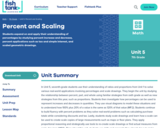Conditional Remix & Share Permitted
CC BY-NC-SA
Rating
0.0 stars

Students expand on and apply their understanding of percentages by studying percent increase and decrease, percent applications such as tax and simple interest, and scaled geometric drawings.

Subject:
Mathematics
Material Type:
Unit of Study
Provider:
Fishtank Learning
Provider Set:
Mathematics
11/19/2021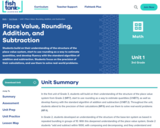Conditional Remix & Share Permitted
CC BY-NC-SA
Rating
0.0 stars

Students build on their understanding of the structure of the place value system, start to use rounding as a way to estimate quantities, and develop fluency with the standard algorithm of addition and subtraction. Students focus on the precision of their calculations, and use them to solve real-world problems.

Subject:
Mathematics
Material Type:
Unit of Study
Provider:
Fishtank Learning
Provider Set:
Mathematics
11/19/2021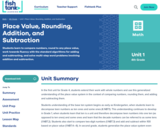Conditional Remix & Share Permitted
CC BY-NC-SA
Rating
0.0 stars

Students learn to compare numbers, round to any place value, work towards fluency with the standard algorithms for adding and subtracting, and solve multi-step word problems involving addition and subtraction.

Subject:
Mathematics
Material Type:
Unit of Study
Provider:
Fishtank Learning
Provider Set:
Mathematics
11/19/2021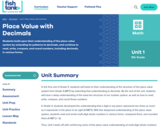Conditional Remix & Share Permitted
CC BY-NC-SA
Rating
0.0 stars

Students build upon their understanding of the place value system by extending its patterns to decimals, and continue to read, write, compare, and round numbers, including decimals, in various forms.

Subject:
Mathematics
Material Type:
Unit of Study
Provider:
Fishtank Learning
Provider Set:
Mathematics
11/19/2021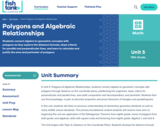Conditional Remix & Share Permitted
CC BY-NC-SA
Rating
0.0 stars

Students connect algebra to geometric concepts with polygons as they explore the distance formula, slope criteria for parallel and perpendicular lines, and learn to calculate and justify the area and perimeter of polygons.

Subject:
Geometry
Mathematics
Material Type:
Unit of Study
Provider:
Fishtank Learning
Provider Set:
Mathematics
11/19/2021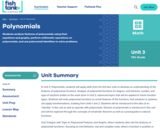Conditional Remix & Share Permitted
CC BY-NC-SA
Rating
0.0 stars

Students analyze features of polynomials using their equations and graphs, perform arithmetic operations on polynomials, and use polynomial identities to solve problems.

Subject:
Algebra
Mathematics
Material Type:
Unit of Study
Provider:
Fishtank Learning
Provider Set:
Mathematics
11/19/2021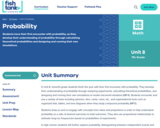Conditional Remix & Share Permitted
CC BY-NC-SA
Rating
0.0 stars

Students have their first encounter with probability, as they develop their understanding of probability through calculating theoretical probabilities and designing and running their own simulations.

Subject:
Mathematics
Material Type:
Unit of Study
Provider:
Fishtank Learning
Provider Set:
Mathematics
11/19/2021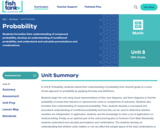Conditional Remix & Share Permitted
CC BY-NC-SA
Rating
0.0 stars

Students formalize their understanding of compound probability, develop an understanding of conditional probability, and understand and calculate permutations and combinations.

Subject:
Geometry
Mathematics
Material Type:
Unit of Study
Provider:
Fishtank Learning
Provider Set:
Mathematics
11/19/2021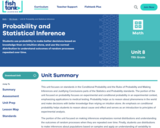Conditional Remix & Share Permitted
CC BY-NC-SA
Rating
0.0 stars

Students use probability to make better decisions based on knowledge than on intuition alone, and use the normal distribution to understand outcomes of random processes repeated over time.

Subject:
Algebra
Mathematics
Material Type:
Unit of Study
Provider:
Fishtank Learning
Provider Set:
Mathematics
11/19/2021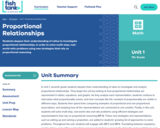Conditional Remix & Share Permitted
CC BY-NC-SA
Rating
0.0 stars

Students deepen their understanding of ratios to investigate proportional relationships, in order to solve multi-step, real-world ratio problems using new strategies that rely on proportional reasoning.

Subject:
Mathematics
Material Type:
Unit of Study
Provider:
Fishtank Learning
Provider Set:
Mathematics
11/19/2021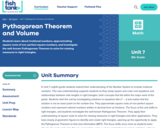Conditional Remix & Share Permitted
CC BY-NC-SA
Rating
0.0 stars

Students learn about irrational numbers, approximating square roots of non-perfect square numbers, and investigate the well-known Pythagorean Theorem to solve for missing measures in right triangles.

Subject:
Mathematics
Material Type:
Unit of Study
Provider:
Fishtank Learning
Provider Set:
Mathematics
11/19/2021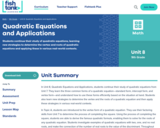Conditional Remix & Share Permitted
CC BY-NC-SA
Rating
0.0 stars

Students continue their study of quadratic equations, learning new strategies to determine the vertex and roots of quadratic equations and applying these in various real-world contexts.

Subject:
Algebra
Mathematics
Material Type:
Unit of Study
Provider:
Fishtank Learning
Provider Set:
Mathematics
11/19/2021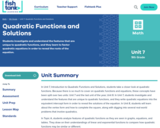Conditional Remix & Share Permitted
CC BY-NC-SA
Rating
0.0 stars

Students investigate and understand the features that are unique to quadratic functions, and they learn to factor quadratic equations in order to reveal the roots of the equation.

Subject:
Algebra
Mathematics
Material Type:
Unit of Study
Provider:
Fishtank Learning
Provider Set:
Mathematics
11/19/2021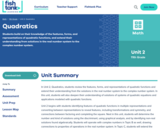Conditional Remix & Share Permitted
CC BY-NC-SA
Rating
0.0 stars

Students build on their knowledge of the features, forms, and representations of quadratic functions, and extend their understanding from solutions in the real number system to the complex number system.

Subject:
Algebra
Mathematics
Material Type:
Unit of Study
Provider:
Fishtank Learning
Provider Set:
Mathematics
11/19/2021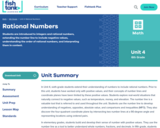Conditional Remix & Share Permitted
CC BY-NC-SA
Rating
0.0 stars

Students are introduced to integers and rational numbers, extending the number line to include negative values, understanding the order of rational numbers, and interpreting them in context.

Subject:
Mathematics
Material Type:
Unit of Study
Provider:
Fishtank Learning
Provider Set:
Mathematics
11/19/2021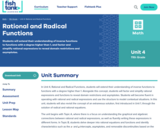Conditional Remix & Share Permitted
CC BY-NC-SA
Rating
0.0 stars

Students will extend their understanding of inverse functions to functions with a degree higher than 1, and factor and simplify rational expressions to reveal domain restrictions and asymptotes.

Subject:
Algebra
Mathematics
Material Type:
Unit of Study
Provider:
Fishtank Learning
Provider Set:
Mathematics
11/19/2021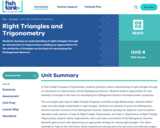Conditional Remix & Share Permitted
CC BY-NC-SA
Rating
0.0 stars

Students develop an understanding of right triangles through an introduction to trigonometry, building an appreciation for the similarity of triangles as the basis for developing the Pythagorean theorem.

Subject:
Geometry
Mathematics
Material Type:
Unit of Study
Provider:
Fishtank Learning
Provider Set:
Mathematics
11/19/2021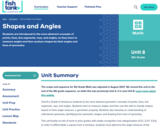Conditional Remix & Share Permitted
CC BY-NC-SA
Rating
0.0 stars

Students are introduced to the more abstract concepts of points, lines, line segments, rays, and angles, as they learn to measure angles and then analyze shapes by their angles and lines of symmetry.

Subject:
Mathematics
Material Type:
Unit of Study
Provider:
Fishtank Learning
Provider Set:
Mathematics
11/19/2021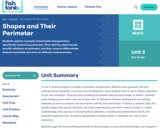Conditional Remix & Share Permitted
CC BY-NC-SA
Rating
0.0 stars

Students explore concepts of perimeter and geometry, specifically measuring perimeter. They start by exploring the specific attribute of perimeter, and then come to differentiate between perimeter and area as different measurements.

Subject:
Mathematics
Material Type:
Unit of Study
Provider:
Fishtank Learning
Provider Set:
Mathematics
11/19/2021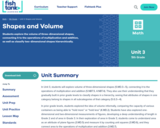Conditional Remix & Share Permitted
CC BY-NC-SA
Rating
0.0 stars

Students explore the volume of three-dimensional shapes, connecting it to the operations of multiplication and addition, as well as classify two-dimensional shapes hierarchically.

Subject:
Mathematics
Material Type:
Unit of Study
Provider:
Fishtank Learning
Provider Set:
Mathematics